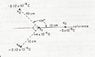# Magnitude of Force

Bama
Ok, here is what I have. See attachment to complete the problem.

What is the magnitude of force exerted on charge q1 by charge q3 and horizontal component of force exerted?

Formula: q1q2 / 4piEor^2

Part 1) (4*10^-6)(2.12*10^-6) / 12.56 (8.85*10^-12)(1*10^-2) = 7.628917917176 N or Rounded off as 7.63N

Part 2) 7.63 N sin 135 degrees = 5.39 N

Now I understand the above, howver what I can understand is the following. The resultant force exerted on the charge q1 by the other 3 charges is what? But what if we replaced q1 with charge -4*10^-6, what resultant force would be exerted by the other 3 charges?

#### Attachments

•Q1 & q2.JPG
17.7 KB · Views: 440

Corneo
For part 1, your answer should be negative since the force is attractive. As for part 2, calculate the vector forces exerted by all the other charges and sum them all up to find the resultant force. If q1 is replaced by a -4*10^-6 C, then the partial forces you calculated to find the resultant force should all change signs.

Bama
Corneo if my answer suppose to be negative by force of attractive, than I am confused. The choices that I have doesn't have a negative solution at all. So is my equation set up incorrctly and if so, how should it be set up?

Corneo
Your formula is correct but you forgot to inclue the sign of q3 in your calculation.

Recall that the electrical force between two charges is given by:
$$F = \frac {1}{4 \pi \epsilon_0} \frac {Q_1Q_2}{r^2}$$

So for part 1) Q1 = 4x10^-6 C and Q2 = -2.12x10^-6 C.

Perhaps the choices for the possible solutions are wrong. Unless the question meant the unsigned magnitude of the force.

Bama
The original question is follow. "What is the magnitude of force exerted on charge q1 by charge q2?

choices: 1) 0 N 3) 3.77 N 5) 7.63 N
2) 0.108 N 4) 5.39 N 6) 10.8 N

The question I am confused about is : "What is the resultant force exerted on charge q1, by the other 3 charges? ( Hint: Note angles carefully)." It also states to selected my answer from one of the above choices.

Also you have Q2 as equaling -2.12x10^-6 C, but as I look at the image only q3 and q4 is eqaul to -2.12x10^-6 C and q2 is equal to -3 x 10^-6. Am I mis-understanding what you meant for your Q2 above?

Corneo
Well I used $Q_1 \text{ and } Q_2$ as variables in general for the formula. Applied in this case, $Q_1 = q_1$ and $Q_2 = q_3$. To answer you last question you can use vector addition to find the resultant force. The formula is

$$\vec F = \frac {1}{4 \pi \epsilon_0} \frac {Q_1Q_2}{r^2} {\vec r}$$

However you could also just calculate the magnitudes of each force exerted on q1, then calculate the components of each vector and sum them all up.